The Central Limit Theorem

# 37 Finite Population Correction Factor

We saw that the sample size has an important effect on the variance and thus the standard deviation of the sampling distribution. Also of interest is the proportion of the total population that has been sampled. We have assumed that the population is extremely large and that we have sampled a small part of the population. As the population becomes smaller and we sample a larger number of observations the sample observations are not independent of each other. To correct for the impact of this, the Finite Correction Factor can be used to adjust the variance of the sampling distribution. It is appropriate when more than 5% of the population is being sampled and the population has a known population size. There are cases when the population is known, and therefore the correction factor must be applied. The issue arises for both the sampling distribution of the means and the sampling distribution of proportions. The Finite Population Correction Factor for the variance of the means shown in the standardizing formula is: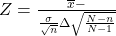and for the variance of proportions is: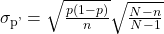The following examples show how to apply the factor. Sampling variances get adjusted using the above formula.

It is learned that the population of White German Shepherds in the USA is 4,000 dogs, and the mean weight for German Shepherds is 75.45 pounds. It is also learned that the population standard deviation is 10.37 pounds.

If the sample size is 100 dogs, then find the probability that a sample will have a mean that differs from the true probability mean by less than 2 pounds.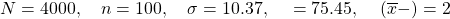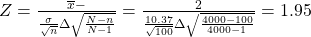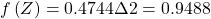Note that “differs by less” references the area on both sides of the mean within 2 pounds right or left.

When a customer places an order with Rudy’s On-Line Office Supplies, a computerized accounting information system (AIS) automatically checks to see if the customer has exceeded his or her credit limit. Past records indicate that the probability of customers exceeding their credit limit is .06.

Suppose that on a given day, 3,000 orders are placed in total. If we randomly select 360 orders, what is the probability that between 10 and 20 customers will exceed their credit limit?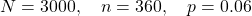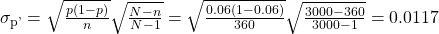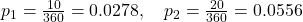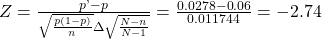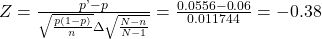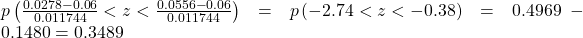A fishing boat has 1,000 fish on board, with an average weight of 120 pounds and a standard deviation of 6.0 pounds. If sample sizes of 50 fish are checked, what is the probability the fish in a sample will have mean weight within 2.8 pounds the true mean of the population?

0.999

An experimental garden has 500 sunflowers plants. The plants are being treated so they grow to unusual heights. The average height is 9.3 feet with a standard deviation of 0.5 foot. If sample sizes of 60 plants are taken, what is the probability the plants in a given sample will have an average height within 0.1 foot of the true mean of the population?

0.901

A company has 800 employees. The average number of workdays between absence for illness is 123 with a standard deviation of 14 days. Samples of 50 employees are examined. What is the probability a sample has a mean of workdays with no absence for illness of at least 124 days?

0.301

Cars pass an automatic speed check device that monitors 2,000 cars on a given day. This population of cars has an average speed of 67 miles per hour with a standard deviation of 2 miles per hour. If samples of 30 cars are taken, what is the probability a given sample will have an average speed within 0.50 mile per hour of the population mean?

0.832

A town keeps weather records. From these records it has been determined that it rains on an average of 37% of the days each year. If 30 days are selected at random from one year, what is the probability that at least 5 and at most 11 days had rain?

0.483

A maker of yardsticks has an ink problem that causes the markings to smear on 4% of the yardsticks. The daily production run is 2,000 yardsticks. What is the probability if a sample of 100 yardsticks is checked, there will be ink smeared on at most 4 yardsticks?

0.500

A school has 300 students. Usually, there are an average of 21 students who are absent. If a sample of 30 students is taken on a certain day, what is the probability that at most 2 students in the sample will be absent?

0.502

A college gives a placement test to 5,000 incoming students each year. On the average 1,213 place in one or more developmental courses. If a sample of 50 is taken from the 5,000, what is the probability at most 12 of those sampled will have to take at least one developmental course?

0.519

### Homework

A company has 1,000 employees. The average number of workdays between absence for illness is 80 with a standard deviation of 11 days. Samples of 80 employees are examined. What is the probability a sample has a mean of workdays with no absence for illness of at least 78 days and at most 84 days?

0.955

Trucks pass an automatic scale that monitors 2,000 trucks. This population of trucks has an average weight of 20 tons with a standard deviation of 2 tons. If a sample of 50 trucks is taken, what is the probability the sample will have an average weight within one-half ton of the population mean?

0.927

A town keeps weather records. From these records it has been determined that it rains on an average of 12% of the days each year. If 30 days are selected at random from one year, what is the probability that at most 3 days had rain?

0.648

A maker of greeting cards has an ink problem that causes the ink to smear on 7% of the cards. The daily production run is 500 cards. What is the probability that if a sample of 35 cards is checked, there will be ink smeared on at most 5 cards?

0.101

A school has 500 students. Usually, there are an average of 20 students who are absent. If a sample of 30 students is taken on a certain day, what is the probability that at least 2 students in the sample will be absent?

0.273